Next: Outer Product Up: Fundamental Concepts Previous: Bra Space

# Operators

We have seen that a functional is a machine that inputs a ket vector and spits out a complex number. Consider a somewhat different machine that inputs a ket vector and spits out another ket vector in a deterministic fashion. Mathematicians call such a machine an operator. We are only interested in operators that preserve the linear dependencies of the ket vectors upon which they act. Such operators are termed linear operators. Consider an operator labeled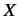. Suppose that when this operator acts on a general ket vector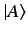it spits out a new ket vector which is denoted. Operatoris linear provided that(25)

for all ket vectorsand, and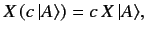(26)

for all complex numbers. Operatorsand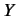are said to be equal if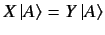(27)

for all kets in the ket space in question. Operatoris termed the null operator if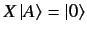(28)

for all ket vectors in the space. Operators can be added together. Such addition is defined to obey a commutative and associate algebra: i.e.,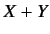(29)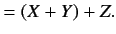(30)

Operators can also be multiplied. The multiplication is associative:(31)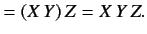(32)

However, in general, it is non-commutative: i.e.,(33)

So far, we have only considered linear operators acting on ket vectors. We can also give a meaning to their operation on bra vectors. Consider the inner product of a general bra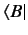with the ket. This product is a number that depends linearly on. Thus, it may be considered to be the inner product ofwith some bra. This bra depends linearly on, so we may look on it as the result of some linear operator applied to. This operator is uniquely determined by the original operator, so we might as well call it the same operator acting on. A suitable notation to use for the resulting bra whenoperates onis. The equation which defines this vector is(34)

for anyand. The triple product of,, andcan be written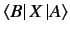without ambiguity, provided we adopt the convention that the bra vector always goes on the left, the operator in the middle, and the ket vector on the right.

Consider the dual bra to. This bra depends antilinearly onand must therefore depend linearly on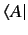. Thus, it may be regarded as the result of some linear operator applied to. This operator is termed the adjoint of, and is denoted. Thus,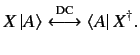(35)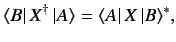(36)

plus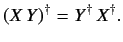(37)

It is also easily seen that the adjoint of the adjoint of a linear operator is equivalent to the original operator. An Hermitian operator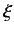has the special property that it is its own adjoint: i.e.,(38)Next: Outer Product Up: Fundamental Concepts Previous: Bra Space
Richard Fitzpatrick 2013-04-08